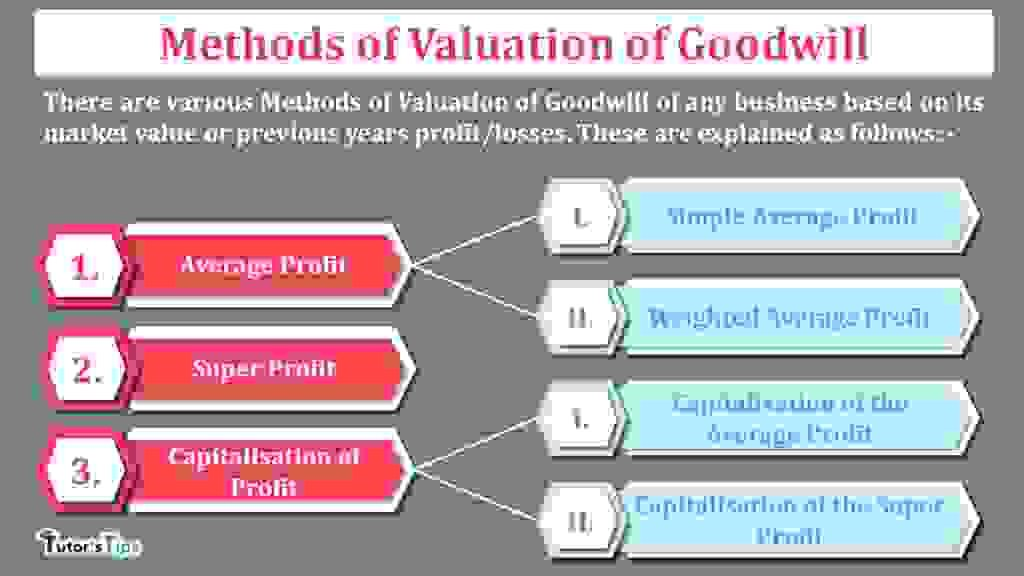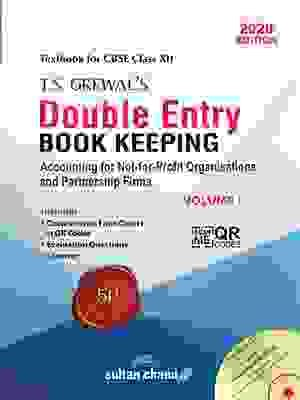# Methods of Valuation of Goodwill – Explained with illustrationsThere are various Methods of Valuation of Goodwill of any business based on its market value or previous years profit/losses. These are explained as follows: –

The Content covered in this article:

## What is the Valuation of Goodwill?

Every aged business has brand value, the faith of customers and satisfaction of employees. Any business who wants to purchase another business it has to pay some amount for all these above factors, this extra amount is known as goodwill. So at the time of selling and buying the business has to calculate the valuation of goodwill. There is a various method to calculate the value of Goodwill which are explained in this article as follows:

## Methods of Valuation of Goodwill: –

There are various Methods of Valuation of Goodwill. Its wholly depend on the owner(partners) that which method they chose to use or as per the partnership deed. all these methods are explained as follows:-

### Average Profit Method: –

Average profit means the average of profits earned in the specific numbers of previous years.

In this method, we will calculate goodwill based on the average of previous years profits. We can calculate two types of average profits i.e. simple average and weighted average. These both are explained as follows: –

#### Simple Average Profit Method: –

Under this method, We have to calculate the simple average profit for the specific number of the years, while calculating the goodwill of the business. Then we have to multiply this amount of profit with the specific number of years of purchases. We have to make adjustments in the profits of all abnormal items included in the profit/loss on the sale of fixed assets.

##### The formula of Simple average profit: –
 Simple Average profit = Total Profit of the n number of year total number of year
##### The formula of Goodwill under Simple average profit method: –
 Goodwill = Simple Average Profit X Number of the years of purchase
##### Illustration 1:

A&B co. ltd. decided to the purchased business of C&D co. ltd. the profit of the last 4 years are shown as the following: –

• 2016 – 25,000
• 2017 – 35,000
• 2018 – 30,000
• 2019 – 50,000

Calculate the goodwill on the base of 3-year purchase of the average profit for the last 4 years.

###### Solution: –

Total Profit of C&D for the last 4 years = 25,000 + 35,000 + 30,000 + 50,000

= 1,40,000/-

 Simple Average profit = Total Profit of the n number of year total number of year

 Simple Average profit = 1,40,000 4

Simple Average profit = 35,000

 Goodwill = Simple Average Profit X Number of the years of purchase
 Goodwill = 35,000 X 3

= 1,05,000

#### Weighted Average Profit Method: –

Under this method, we have to calculate the weighted average profit for a specific number of the years while calculating the goodwill of the business. Weighted average profit is calculated by multiplying the amount of adjusted profit with the specific weight. Then we have to multiply this amount of profit with the specific number of years of purchases. We have to make adjustments in the profits of all abnormal items included in the profit/loss on the sale of fixed assets.

##### The formula of Weighted average profit: –
 Weighted Average profit = Sum of Profits multiplied by weights Sum of weights
##### The formula of Goodwill under Weighted average profit method: –
 Goodwill = Weighted Average Profit X Number of the years of purchase
##### Illustration 2:

A&B co. ltd. decided to the purchased business of C&D co. ltd. the profit of the last 4 years are shown as the following: –

• 2016 – 25,000
• 2017 – 35,000
• 2018 – 30,000
• 2019 – 50,000

Calculate the goodwill on the base of 3-year purchase of the Weighted average profit for the last 4 years.

###### Solution: –
 Year Profit Weight Weighted Profit (Rs) I II III IV = II * III 2016 25,000 1 25,000 2017 35,000 2 70,000 2018 30,000 3 90,000 2019 50,000 4 2,00,000 Total 10 3,85,000

Sum of Profits multiplied by weights = 3,85,000/-

 Weighted Average profit = Sum of Profits multiplied by weights Sum of weights
 Weighted Average profit = 3,85,000 10

Weighted Average profit = 38,500

 Goodwill = Weighted Average Profit X Number of the years of purchase
 Goodwill = 38,500 X 3

Goodwill  = 1,15,500/-

### Super Profit Method: –

Super Profits means an excess amount of average profit over the normal profit (which is normally or easily earned by the same type of other business in the industry).

Under this method, We have to calculate the super profit of the business for the specific number of the years, while calculating the goodwill of the business. Super profit is calculated by subtracting the total actual/average profit of the specific year from the normal profit earned by the same type of firm in the industry. Then we have to multiply this amount of profit with the specific number of years of purchases. We have to make adjustments in the profits of all abnormal items included in the profit/loss on the sale of fixed assets.

#### The formula of Super profit: –

 Super profit = Actual/Average Profit – Normal Profit

#### The formula of Goodwill under Simple average profit method: –

 Goodwill = Super Profit X Number of the years of purchase

#### Illustration 3:

A&B co. ltd. decided to the purchased business of C&D co. ltd. the profit of the last 4 years are shown as the following: –

• 2016 – 25,000
• 2017 – 35,000
• 2018 – 30,000
• 2019 – 50,000

Calculate the goodwill on the base of the 3-year purchase of the super profit method.  But normal profit earned by the other firms in the industry is equal to 27,500.

##### Solution: –

Total Profit of C&D for the last 4 years = 25,000 + 35,000 + 30,000 + 50,000

= 1,40,000/-

 Simple Average profit = Total Profit of the n number of year total number of year

 Simple Average profit = 1,40,000 4

Average profit = 35,000

 Super profit = Actual/Average Profit – Normal Profit

Super Profit = 35,000 – 27,500

Super Profit = 7,500

 Goodwill = Super Profit X Number of the years of purchase

Goodwill = 7,500 X 3

Goodwill = 22,500/-

### Capitalisation Method:-

We have to capitalise the profits to calculate the amount of goodwill of the business. we can capitalise both types of profits i.e. super profit or normal profit, these are sub-methods of capitalisation method of goodwill. These Methods of Valuation of Goodwill are shown as below: –

#### The capitalisation of the Average Profit Method: –

In this method, first, we have to calculate the simple average profit of the business for the specifics years and then we will multiply it with the 100 and divide with an average rate of return. This is also known as business valuation.

After that, we will calculate the net assets of the business. then to calculate the goodwill we have to subtract the total value of net assets from the capitalised average profit.

#### The formula of Capitalisation of Average profit/ Value of business: –

 Capitalisation of Average profit = Average Profit X 100 Normal Rate of Return

#### The formula of Net assets: –

 Net assets = Total Assets – Outsiders’ liabilities

Note: – Total Assets does not include the goodwill, non-trade investment and fictitious assets

#### The formula of Goodwill under Capitalisation of average profit method: –

 Goodwill = Capitalisation of Average profit – Net assets

#### Illustration 4:

A&B co. ltd. decided to the purchased business of C&D co. ltd. the average profit of the last 4 years is 35,000. Net assets of the firms are Rs 3,00,000. The normal rate of return is 10%.

Calculate the goodwill by the capitalisation of average profit method.

##### Solution: –
 Capitalisation of Average profit = Average Profit X 100 Normal Rate of Return
 Capitalisation of Average profit = 35,000 X 100 10

Capitalisation of Average profit = 3,50,000

 Goodwill = Capitalisation of Average profit – Net assets
 Goodwill = 3,50,000 – 3,00,000

Goodwill = 50,000/-

#### The capitalisation of the Super Profit Method: –

In this method, first, we have to calculate the super profit of the business for the specifics years and then we will multiply it with the 100 and divide with a normal rate of return, this amount is the amount of goodwill.

But to calculate the amount of super profit we have to calculate the amount of capital employed/Net Assets and normal profit.

#### The formula of Goodwill under Capitalisation of super profit method: –

 Goodwill = Super Profit X 100 Normal Rate of Return

#### The formula of Capital Employed/Net assets: –

 Net assets = Total Assets – Outsiders’ liabilities

Note: – Total Assets does not include the goodwill, non-trade investment and fictitious assets

#### Illustration 5:

A&B co. ltd. decided to the purchased business of C&D co. ltd. the Actual average profit of the last 4 years is 40,000. Total assets of the firms are Rs 4,00,000 and outsiders’ liabilities are Rs 1,00,000. The normal rate of return is 10%.

Calculate the goodwill by the capitalisation of average profit method.

##### Solution: –

Calculate the to Capital Employed/ Net Assets: –

Net Asset =  Total assets – Outsiders’ Liabilities

Net Assets = 4,00,000 – 1,00,000

Net Assets = 3,00,000

Now, Calculate the Normal Profit: –

Normal Profit = Capital Employed X Normal rate of return

Normal Profit = 3,00,000 X 10%

Normal Profit = 30,000

Now, Calculate the Super Profit: –

Super Profit = Actual Profit – Normal Profit

Super Profit = 35,000 – 30,000

Super Profit = 5,000

 Goodwill = Super Profit X 100 Normal Rate of Return
 Goodwill = 5,000 X 100 10

Goodwill  = 50,000/-

Thanks for reading the topic of Methods of Valuation of Goodwill.

please comment your feedback whatever you want. If you have any question please ask us by commenting.+2 Book 1